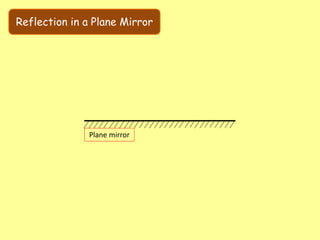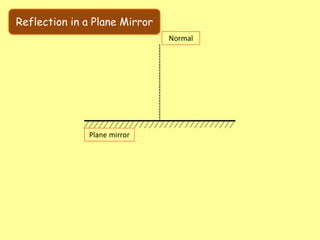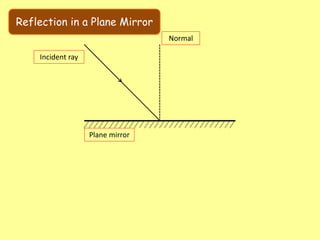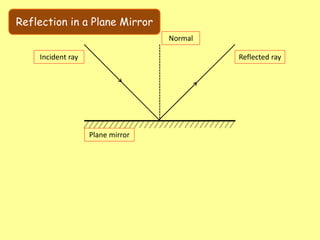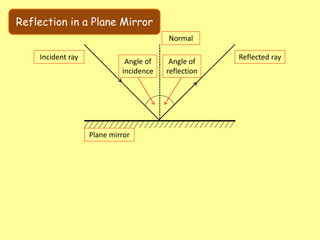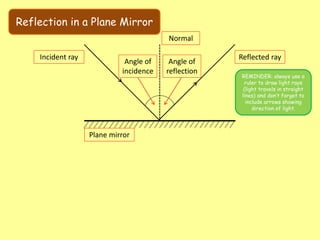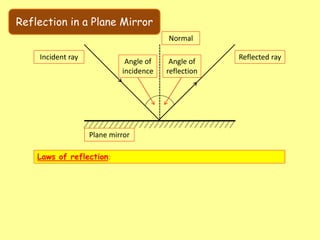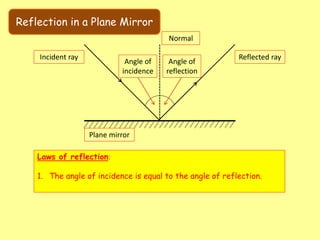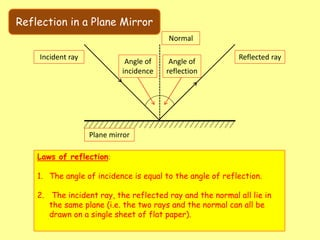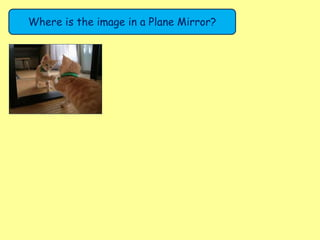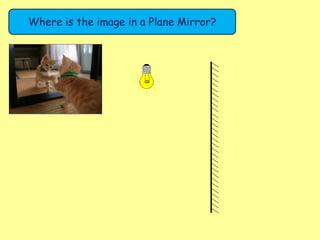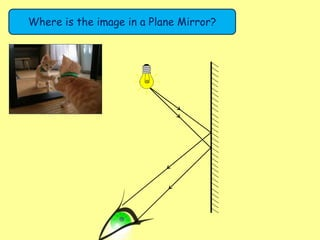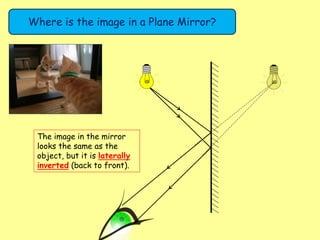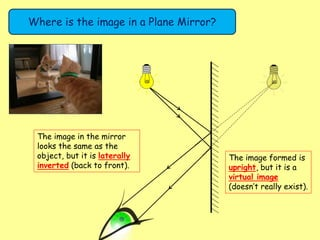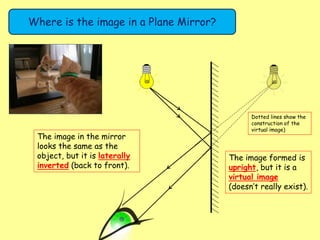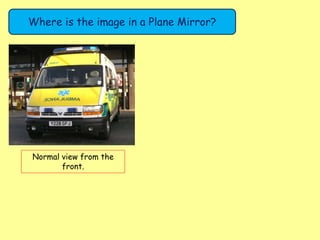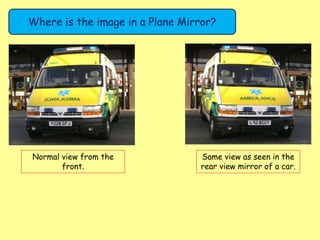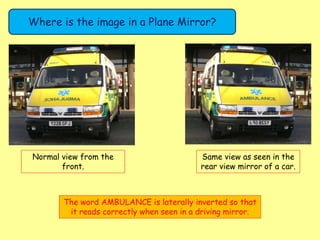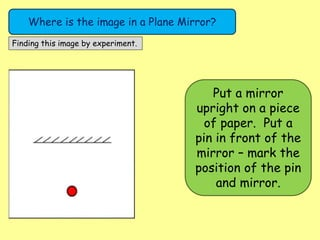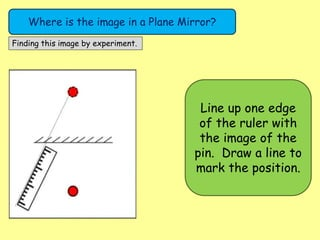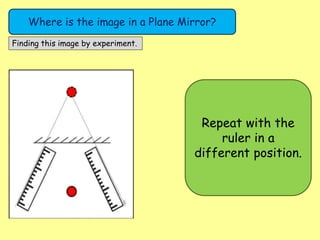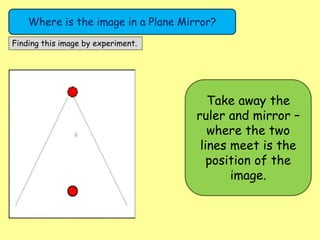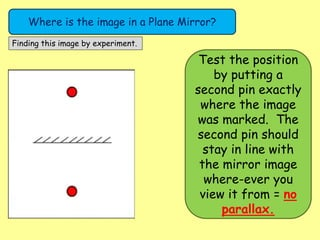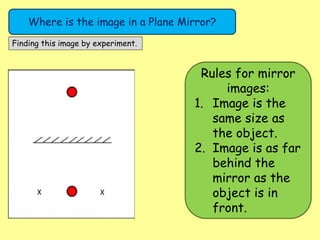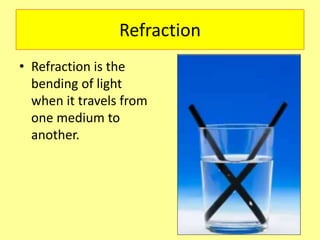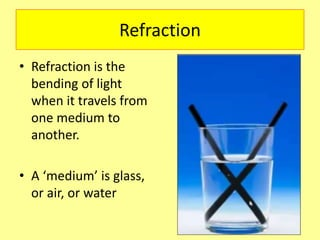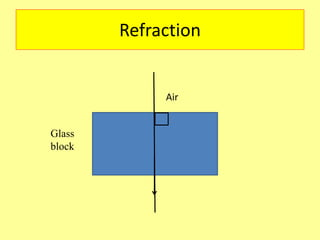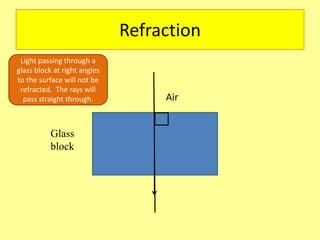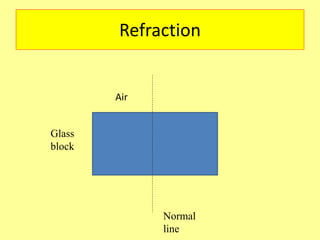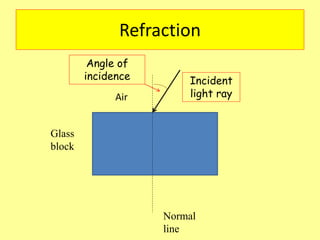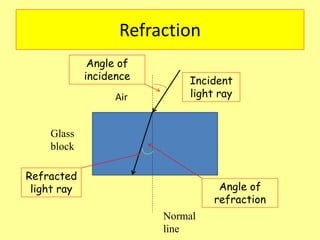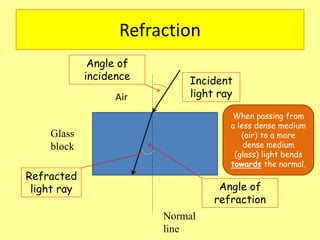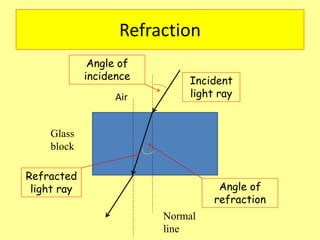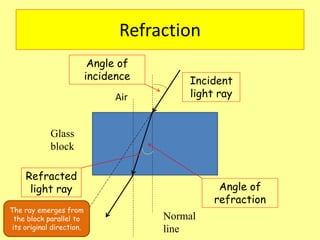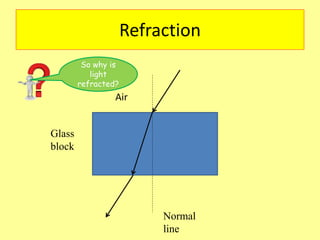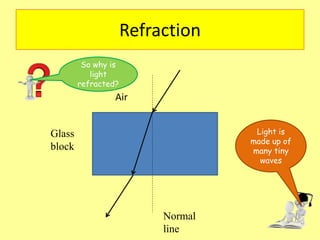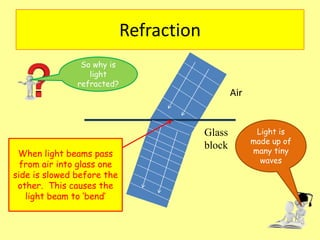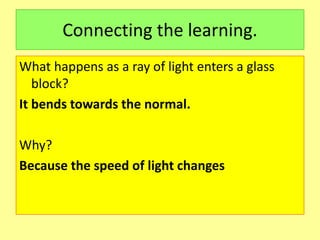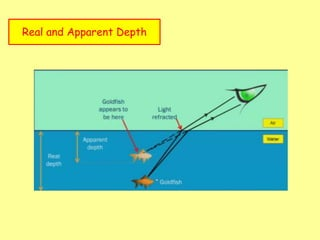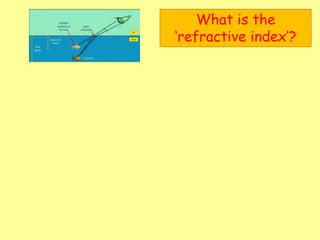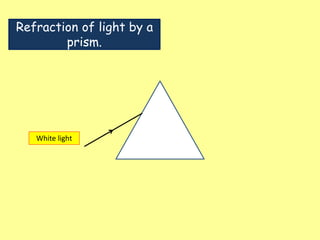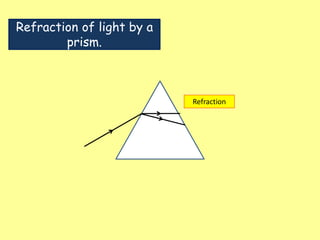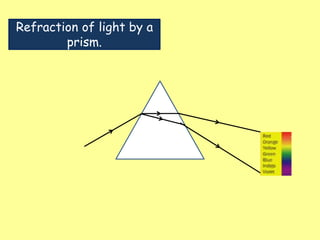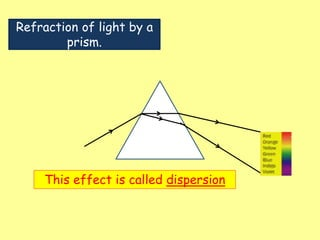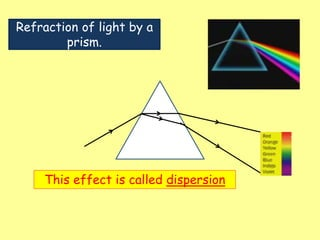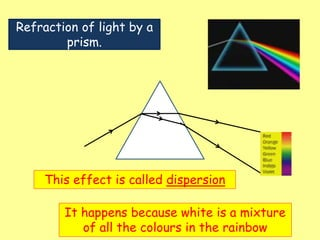1 de 46Light – reflection refractionrahul670905Class 10 sa 2... 2017-2018Anupam NaranglightAnjaliKumari3371 Reflaction Of LightHamsiah DahalanPLANE-MIRRORS.pdfArisJrOrdoez1 Reflaction Of LightHamsiah Dahalan

### Physics 18 - Reflection and Refraction.pptx

1. Reflection in a Plane Mirror Plane mirror
2. Reflection in a Plane Mirror Plane mirror Normal
3. Reflection in a Plane Mirror Plane mirror Normal Incident ray
4. Reflection in a Plane Mirror Plane mirror Normal Incident ray Reflected ray
5. Reflection in a Plane Mirror Plane mirror Normal Incident ray Reflected ray Angle of incidence Angle of reflection
6. Reflection in a Plane Mirror Plane mirror Normal Incident ray Reflected ray Angle of incidence Angle of reflection REMINDER: always use a ruler to draw light rays (light travels in straight lines) and don’t forget to include arrows showing direction of light.
7. Reflection in a Plane Mirror Plane mirror Normal Incident ray Reflected ray Angle of incidence Angle of reflection Laws of reflection:
8. Reflection in a Plane Mirror Plane mirror Normal Incident ray Reflected ray Angle of incidence Angle of reflection Laws of reflection: 1. The angle of incidence is equal to the angle of reflection.
9. Reflection in a Plane Mirror Plane mirror Normal Incident ray Reflected ray Angle of incidence Angle of reflection Laws of reflection: 1. The angle of incidence is equal to the angle of reflection. 2. The incident ray, the reflected ray and the normal all lie in the same plane (i.e. the two rays and the normal can all be drawn on a single sheet of flat paper).
10. Where is the image in a Plane Mirror?
11. Where is the image in a Plane Mirror?
12. Where is the image in a Plane Mirror?
13. Where is the image in a Plane Mirror? The image in the mirror looks the same as the object, but it is laterally inverted (back to front).
14. Where is the image in a Plane Mirror? The image in the mirror looks the same as the object, but it is laterally inverted (back to front). The image formed is upright, but it is a virtual image (doesn’t really exist).
15. Where is the image in a Plane Mirror? The image in the mirror looks the same as the object, but it is laterally inverted (back to front). The image formed is upright, but it is a virtual image (doesn’t really exist). Dotted lines show the construction of the virtual image)
16. Where is the image in a Plane Mirror? Normal view from the front.
17. Where is the image in a Plane Mirror? Normal view from the front. Same view as seen in the rear view mirror of a car.
18. Where is the image in a Plane Mirror? Normal view from the front. Same view as seen in the rear view mirror of a car. The word AMBULANCE is laterally inverted so that it reads correctly when seen in a driving mirror.
19. Where is the image in a Plane Mirror? Finding this image by experiment. Put a mirror upright on a piece of paper. Put a pin in front of the mirror – mark the position of the pin and mirror.
20. Where is the image in a Plane Mirror? Finding this image by experiment. Line up one edge of the ruler with the image of the pin. Draw a line to mark the position.
21. Where is the image in a Plane Mirror? Finding this image by experiment. Repeat with the ruler in a different position.
22. Where is the image in a Plane Mirror? Finding this image by experiment. Take away the ruler and mirror – where the two lines meet is the position of the image.
23. Where is the image in a Plane Mirror? Finding this image by experiment. Test the position by putting a second pin exactly where the image was marked. The second pin should stay in line with the mirror image where-ever you view it from = no parallax.
24. Where is the image in a Plane Mirror? Finding this image by experiment. X X Rules for mirror images: 1. Image is the same size as the object. 2. Image is as far behind the mirror as the object is in front.
25. Refraction • Refraction is the bending of light when it travels from one medium to another.
26. Refraction • Refraction is the bending of light when it travels from one medium to another. • A ‘medium’ is glass, or air, or water
27. Refraction Glass block Air
28. Refraction Glass block Air Light passing through a glass block at right angles to the surface will not be refracted. The rays will pass straight through.
29. Refraction Glass block Normal line Air
30. Refraction Glass block Normal line Incident light ray Air Angle of incidence
31. Refraction Glass block Normal line Incident light ray Air Angle of incidence Angle of refraction Refracted light ray
32. Refraction Glass block Normal line Incident light ray Air Angle of incidence Angle of refraction Refracted light ray When passing from a less dense medium (air) to a more dense medium (glass) light bends towards the normal.
33. Refraction Glass block Normal line Incident light ray Air Angle of incidence Angle of refraction Refracted light ray
34. Refraction Glass block Normal line Incident light ray Air Angle of incidence Angle of refraction Refracted light ray The ray emerges from the block parallel to its original direction.
35. Refraction Glass block Normal line Air So why is light refracted?
36. Refraction Glass block Normal line Air So why is light refracted? Light is made up of many tiny waves
37. Refraction Glass block Air So why is light refracted? Light is made up of many tiny waves When light beams pass from air into glass one side is slowed before the other. This causes the light beam to ‘bend’
38. Connecting the learning. What happens as a ray of light enters a glass block? It bends towards the normal. Why? Because the speed of light changes
39. Real and Apparent Depth
40. What is the ‘refractive index’?
41. Refraction of light by a prism. White light
42. Refraction of light by a prism. Refraction
43. Refraction of light by a prism.
44. Refraction of light by a prism. This effect is called dispersion
45. Refraction of light by a prism. This effect is called dispersion
46. Refraction of light by a prism. This effect is called dispersion It happens because white is a mixture of all the colours in the rainbow